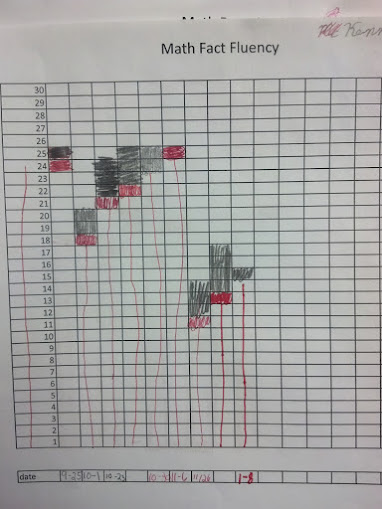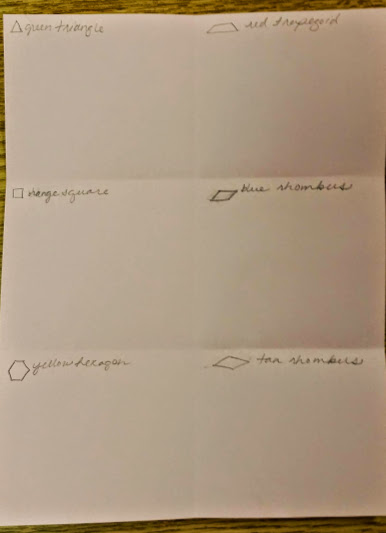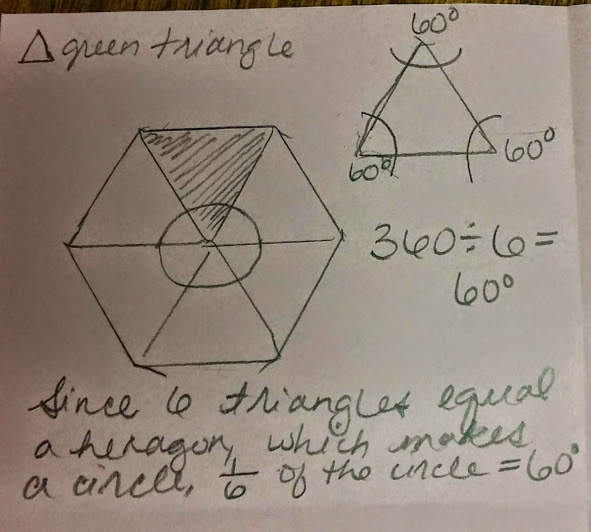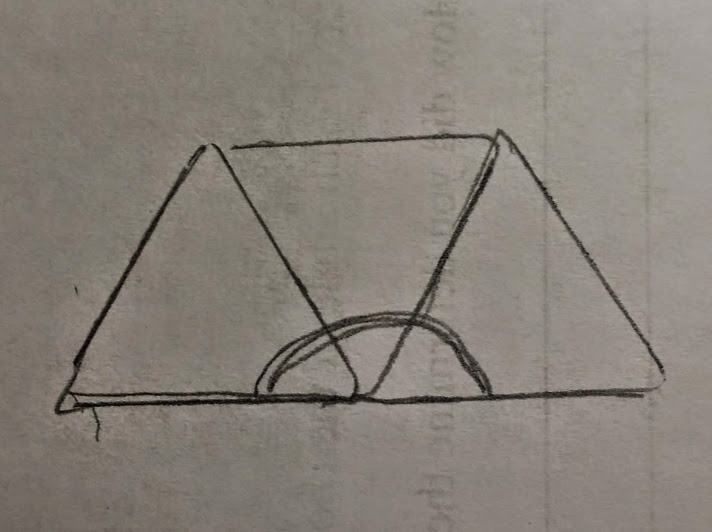# Angles and Pattern Blocks

16 teachers like this lesson
Print Lesson

## Objective

SWBAT find angle measurements of pattern blocks.

#### Big Idea

In this lesson, students work with a partner and pattern blocks to discover angle measurements and properties.

## Warm Up

7 minutes

Students will start today's lesson with a fluency assessment.  This assessment is from Monitoring Basic Skills Progress Second Edition: Basic Math Computation by Lynn S. Fuchs, Carol L. Hamlett, and Douglas Fuchs.

This is an assessment I have my students do each week and then graph their results.  It allows them to reflect on their learning of basic math facts, as well as using all four operations with whole numbers, and adding and subtracting unit fractions.  (It also happens to be the quietest time in my math classroom all week!!)

This is what my classroom looks like as students work on this assessment.

This is sample of a student's graph. You can also click here to hear my thoughts about this graph below.Click hereto see an example of a typical fourth grade fluency decrease since students are completing the fourth grade fluency set that incorporates division, multiplication, addition, subtraction, and adding and subtracting fractions with like denominators. (At this point, students have not had many fraction lessons, thus very very students are able to complete the fraction problems)

I do not start my students with the fourth grade skills, but at this point in the year, all students are using the fourth grade set.. I chose to start them with the end of the third grade skills which covers addition, subtraction and multiplication and division of basic facts. I strongly believe in a balanced math approach, which is one reason why I also believe in common core standards.  By having a balance of building conceptual understanding, application of problems, and computational fluency, students can experience rigorous mathematics.  I want to make clear that this assessment ONLY measures basic math computation.  It is only one piece of students' knowledge. The assessments in this book, for each grade level, do not change in difficulty over the course of the year.  Therefore, a student's increase in score over the school year truly reflects improvement in the student's ability to work the math problems at that grade level.

## Concept Development

45 minutes

Students use hands-on problem solving strategies to determine the measures of the angles of each pattern block. They engage in discussion which allows them to verbalize their own strategies and listen to those of others. Discussion and exploration are the key elements in developing mathematical reasoning

Students need pattern blocks for this lesson: One set of pattern blocks for each student (one each: square, triangle, trapezoid,hexagon, blue parallelogram, tan parallelogram). This activity is designed as an exploration. It works best if the students receive very little direction at the beginning.

To begin this lesson, I first review with students some key angle terms; obtuse angle, acute angle, straight angle, and right angle.  Then I instruct students how to organize their paper for this lesson.  Since I want to have record of students thinking and work, I instruct them to set up their paper like this:Students will trace each shape in the space provided and then label each angle of the shape with the correct angle measurement. They will also write about how her/she determined the measurements.

Next I ask students how they think they would determine the angle measures of each angle of the triangle. (Students know that 6 triangles make a hexagon, and I predicted their responses. The example that follows is based off of this)

Students respond with that they know six triangles make a hexagon. One student comments that a hexagon is "like a circle with points."  We talk briefly about what that means.  Next I draw a circle through six hexagons like this;I then show them how to trace the triangles on their paper in order to "show" their work for today's assignment.  Students trace the six triangles on their paper.  Next, I ask them how to determine the angle measurement of each triangle vertex.  Many students may already know that the triangle is equilateral and has 60 degrees at each vertex, but others do not.  I guide students thinking through questioning and listen to their ideas.  I  then draw a half circle through the triangles like this:Next, I ask students if the semi circle changed their thinking about how to determine the angle measurements.  I lead a brief discussion and allows students to share out their thinking about the angle measures of the green triangle.  Then we fill out the paper together for the triangles.  Next, students work with their learning partners, in the same way we worked to discover the triangle angle measures, and finish the other five shapes. (Note: most of my students know that the square has 90 degree angles. I tell them they must prove it or show it ANOTHER way using picture, numbers, and/or words)

Listen to this student explaining how to find the angle measurements for the blue rhombus.

This student explains how to find the angle measurements of the green triangle.

Once students have had time to discover the measure of each angle, students share their thinking with the rest of the class. This is an important part of this lesson.  During this part of the lesson, I help make connections and with division, circles, and angle measurements.  In the video below you can see a student sharing his ideas during this share and discussion time.